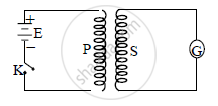Share

# Explain the Phenomenon of Mutual Induction. - Physics

#### Question

Explain the phenomenon of mutual induction.

#### Solution

a.  The phenomenon of production of induced e.m.f in one coil due to change of  current in the neighbouring coil is called mutual induction. The e.m.f so induced is called mutually induced e.m.f.

b.Consider primary coil P and secondary coil S fitted with galvanometer G and placed very close to each other as shown in figure. The coil P is connected in series with the source of e.m.f (battery) and key K.

c. When tap key K is pressed, current IP passes through the coil P. Magnetic flux φS linked with secondary coil S at any instant is directly proportional to current IP through primary coil P at that instant.
∴ φS ∝ IP
∴ φS = M IP ….(i)

where M is constant called coefficient of mutual induction or mutual inductance of the coil.

d.  e.m.f induced in S at any instant is given by,
e_S = -(dphi_s)/(dt)

e_S=-d/(dt)(MI_P) [From equation (i)]

∴  e_S = − M (dI_P)/dt
∴ Magnitude of induced e.m.f is given by,

|e_S|=|-(MdI_P)/dt|

e_s=(MdI_P)/dt

M=e_S/((dI_P)/dt)

Is there an error in this question or solution?